0%

## 自回归模型（AR）

### 概述

• 自回归模型一种时间序列预测模型，是若干随机数的集合。描述的是当前值与历史值得关系，是一种随机过程
• 对于指定值的预测是对于以往值的线性预测加上随机扰动项，所以这个模型的形式是一个随机差分方程
• 是ARMA（Autoregressive–moving-average model）和VAR（Vector Autoregressive model）一种特殊形式
• p阶自回归模型记作AR(p)

AR(p)模型的定义如下

### 序列平稳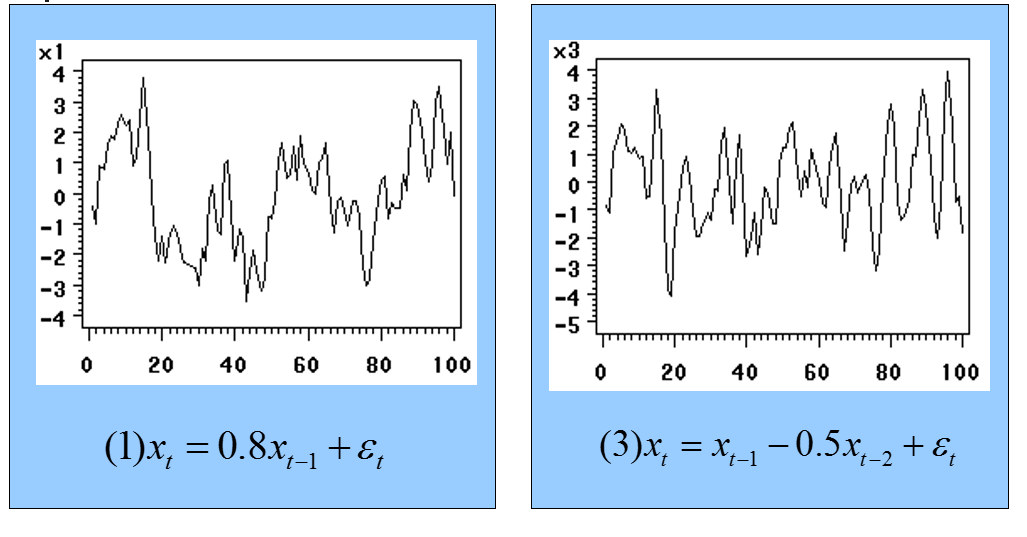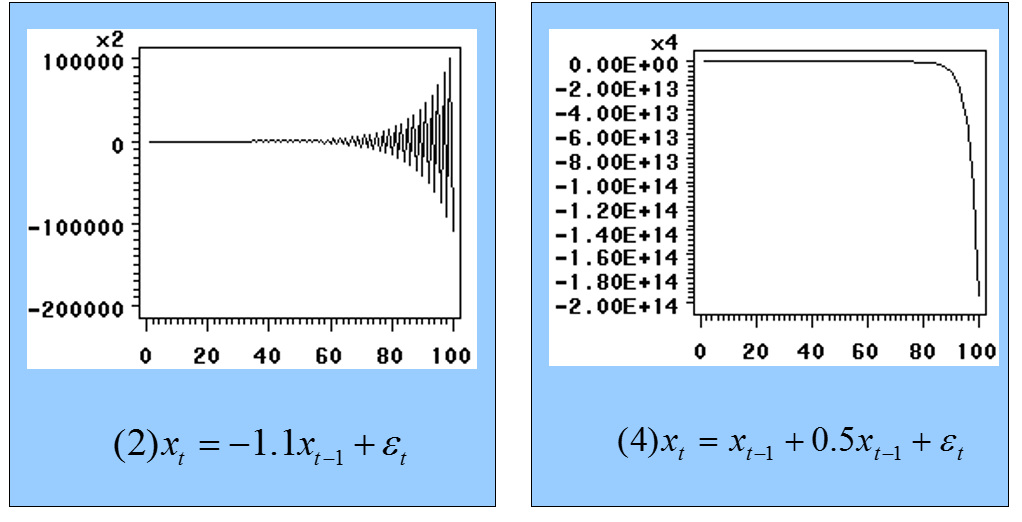• 均值与统计时间无关
• 方差与统计时间无关
• 协方差与统计时间无关，只与统计间隔有关

AR(p)模型平稳的充要条件是回归系数多项式$\phi(B)$的根都在单位圆外

### 模型判定

#### 模型特点

1. 自相关函数拖尾——指数级递减，衰减至0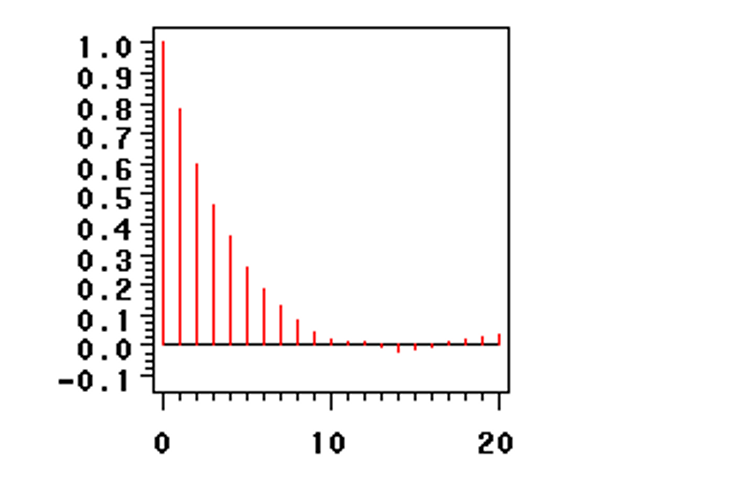2. 偏自相关函数P阶截尾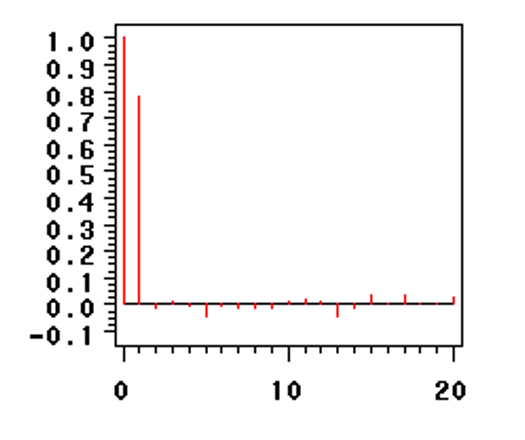### 模型参数求解

#### Yule-Walker估计

\begin{equation}
\begin{bmatrix}
\gamma_1 \\
\gamma_2 \\
\vdots \\
\gamma_p
\end{bmatrix}
=
\begin{bmatrix}
\gamma_0 & \gamma_{-1} & \gamma_{-2} & \cdots \\
\gamma_1 & \gamma_{0} & \gamma_{-2} & \cdots \\
\vdots & \vdots & \vdots & \ddots \\
\gamma_{p-1} & \gamma_{p-2} & \gamma_{p-3} & \cdots \\
\end{bmatrix}
\begin{bmatrix}
\varphi_1 \\
\varphi_2 \\
\vdots \\
\varphi_p
\end{bmatrix}
\nonumber
\end{equation}

## 滑动平均模型（MA）

### 概述

• 滑动平均模型描述的是自回归过程的误差累计
• q阶滑动平均模型记作MA(q)

MA(q)模型的定义如下

### 与自回归模型的白噪声对比

1. 影响方式不同。前几项白噪声直接影响后面的值，而AR模型中前几项的白噪声并没有直接出现在等式右边，只是通过影响前几项的值，然后前几项的值再去间接影响后面的值。
2. 影响范围不同。MA模型的噪声值只能影响当时时刻的值和之后的q个时刻的值，而AR模型的每一项噪声都能对无穷远项产生影响。

### 模型判定

$MA(q)$的自相关系数为

\begin{cases}
(-\theta_k + \theta_1\theta_{k + 1} + \theta_2\theta_{k + 2} + \dots + \theta_{q - k}\theta_{q})\sigma^2 & k = 1, 2, \dots, q \\
0 & k > q
\end{cases}

1. 自相关函数q阶截尾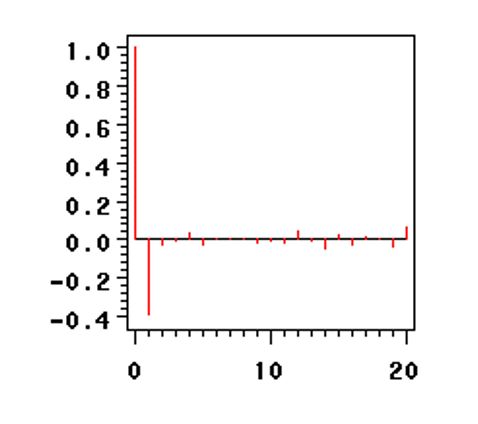2. 偏自相关函数拖尾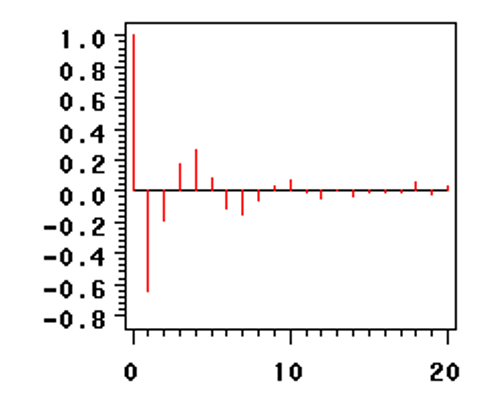## 自回归滑动平均模型（ARMA）

### 概述

• 由自回归模型和滑动平均模型两部分组合而成
• 用于理解甚至预测一组时间序列数据
• p阶自回归模型和q阶滑动回归模型所组合起来的自回归滑动平均模型记作ARMA(p,q)

ARMA(p, q)的定义如下

### 模型参数求解

ARMA(p, q)模型无法通过最小二乘法直接解出，通常的做法是先通过数据选择出最小的p和q

### 模型判定

1. 自相关函数拖尾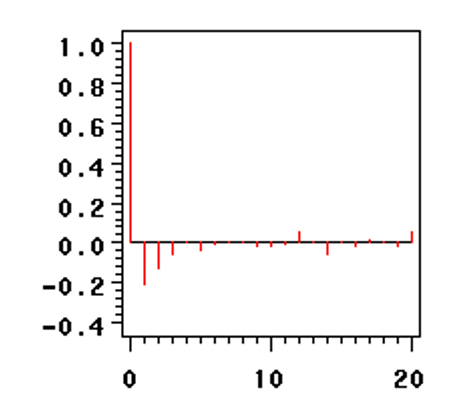2. 偏自相关函数拖尾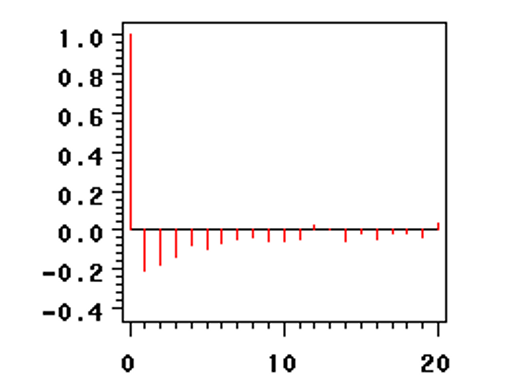## 自回归求和滑动平均模型（ARIMA）

### 概述

• ARIMA全称Autoregressive Integrated Moving Average
• 是ARMA模型的泛化形式
• 适用于数据不平稳的情形
• 通过差分的方式降低数据的不平稳特性然后转化为ARMA模型
• 又称Box-Jenkins法

### 定义

ARIMA(p, d, q)的定义如下

### 模型参数求解

p, d, q中最先确定d，即使序列平稳的差分次数，通常情况下d<3

AR 拖尾 截尾 平稳
MA 截尾 拖尾 平稳
ARMA 拖尾 拖尾 平稳
ARIMA 拖尾 拖尾 不平稳

## 应用步骤

### 白噪声检验

1. 任取$t \in T$有$EX_t = \mu$
2. 任取$t , s \in T$有

\begin{equation}
cov(t, s) =
\begin{cases}
\sigma^2 & t = s \\
0 & t \neq s
\end{cases}
\end{equation
}

### 模型识别

95%置信区间：如果样本(偏)自相关系数在最初的d阶明显大于两倍标准差范围，而后几乎95％的自相关系数都落在2倍标准差的范围以内，而且通常由非零自相关系数衰减为小值波动的过程非常突然。这时，通常视为(偏)自相关系数截尾。截尾阶数为d

### 模型定阶

• 对于简单的ARMA模型通过观察自相关函数和偏相关函数可以进行初步定阶
• 复杂的ARMA模型通过赤池信息量准则定阶